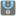• # Level 11 IM STUCK HERE FOREVER

## Question related to missionThe Most Numbers

Let's work with numbers.

You are given an array of numbers (floats). You should find the difference between the maximum and minimum element. Your function should be able to handle an undefined amount of arguments. For an empty argument list, the function should return 0.

Floating-point numbers are represented in computer hardware as base 2 (binary) fractions. So we should check the result with ±0.001 precision. Think about how to work with an arbitrary number of arguments.

Input: An arbitrary number of arguments as numbers (int, float).

Output: The difference between maximum and minimum as a number (int, float).

Precondition: 0 ≤ len(args) ≤ 20 all(-100 < x < 100 for x in args) all(isinstance(x, (int, float)) for x in args)

IVE BEEN STUCK HERE FOREVER

this is the code I need to solve:

def checkio(*args): return 0

# These "asserts" using only for self-checking and not necessary for auto-testing

if name == 'main': def almostequal(checked, correct, significantdigits): precision = 0.1 ** significant_digits return correct - precision < checked < correct + precision

```assert almost_equal(checkio(1, 2, 3), 2, 3), "3-1=2"
assert almost_equal(checkio(5, -5), 10, 3), "5-(-5)=10"
assert almost_equal(checkio(10.2, -2.2, 0, 1.1, 0.5), 12.4, 3), "10.2-(-2.2)=12.4"
assert almost_equal(checkio(), 0, 3), "Empty"
print("Coding complete? Click 'Check' to review your tests and earn cool rewards!")
```7